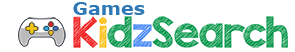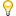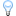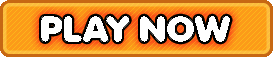Play Fast Facts Basic Math Facts Addition, Subtraction, Multiplication, Division | Free Online Games | KidzSearch.com# Fast Facts Basic Math Facts Addition, Subtraction, Multiplication, Division

5 stars (1,129 plays / 1 votes)Fast Facts Basic Math Facts Addition, Subtraction, Multiplication, DivisionHow quickly can you correctly answer 10 math facts
5 stars out of 5 (1,129 plays / 1 votes)

KidzSearch > Games > Math Games
instructions · reload · report · helpTags:  math    basic    facts    addition    subtraction    multiplication    division    problems    elementary    number    sense    first    timed    test    quiz    divide    multiply
 Find KidzSearch Games   kidzsearch   all sites  KidzSearch > Games > Math GamesMember of the American Library Association  Copyright 2005-2021 KidzSearch.com